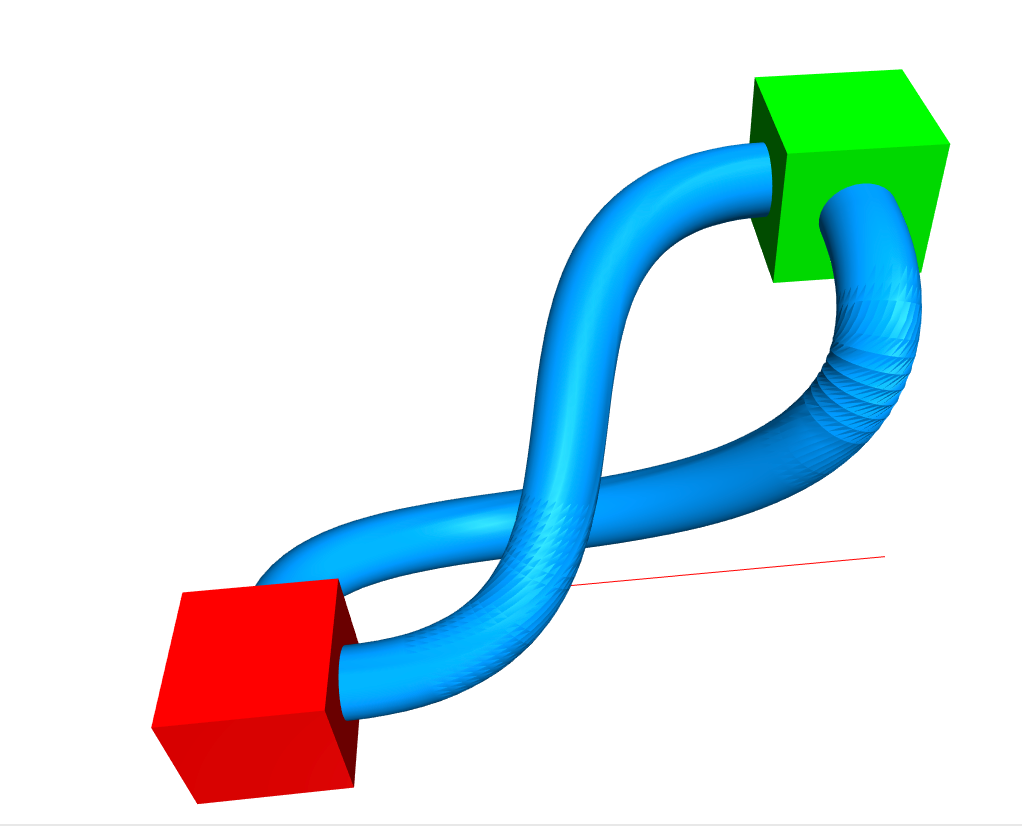# Bezier tubes

•Updated my bezier implementation, and I wanted to share. :).

It can now handle an arbitrary number of control points, and an arbitrary number of dimensions. Also allows for getting the tangential vector at any point along the curve.

``````tube([
[8,2,12],
[8,-6,12],
[8,0,0],
[-8,4,2],
[-8,-2,2]
]);

function tube(bezierControlPoints) {
let circ = circle({radius: 1, segments:32});
let circPoints = geometry.geom2.toPoints(circ);
let tubeSlice = slice.fromPoints(circPoints);

var tubeCurve = math.bezier.create(bezierControlPoints);

let tube = extrusions.extrudeFromSlices({
numberOfSlices: 60,
isCapped: true,
callback: function (progress, count, base) {
let position = tubeCurve.at(progress);
let tangent = tubeCurve.tangentAt(progress);
let rotation = fromVectors(math.vec3.fromArray([0,0,1]),math.vec3.fromArray(tangent));
let translation = math.mat4.fromTranslation(position);
let newslice = slice.transform(math.mat4.multiply(translation, rotation), base);
return newslice
}
}, tubeSlice);
return tube;
}

``````

My rotation matrix from vectors code is a little funky when it's near 180 degrees, but otherwise, pretty happy with it.

• @SimonClark this is cool. thanks for sharing.

FYI, this functionality will behave far better after the next update, which folds full numeric precision back into the library. (And 10X improved speed is included for free!)